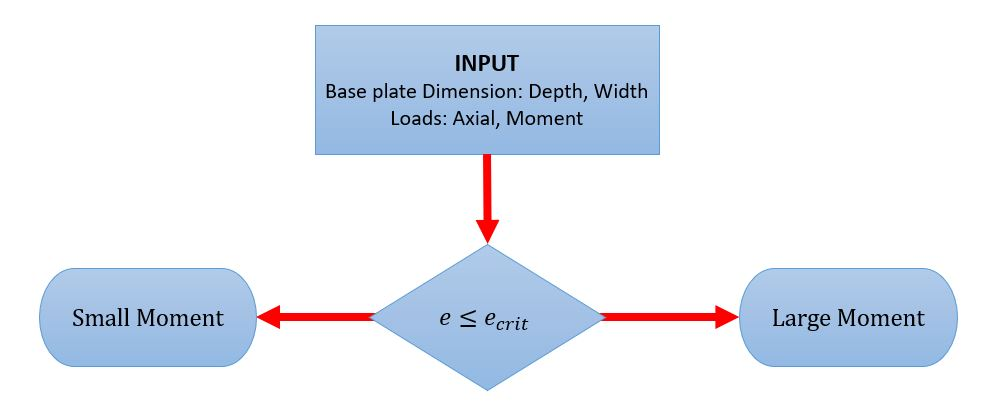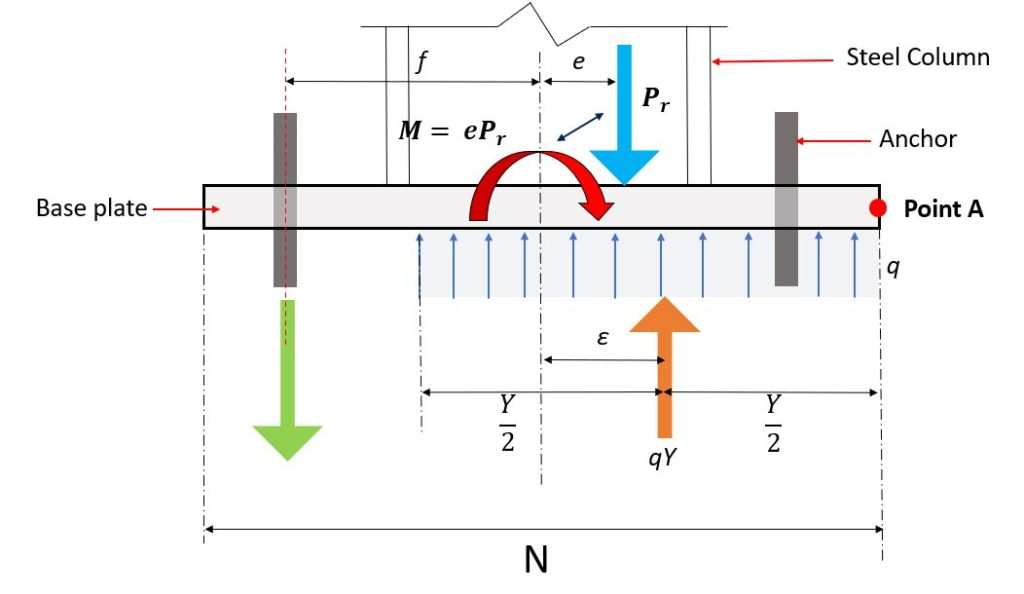SkyCiv Documentation

Your guide to SkyCiv software - tutorials, how-to guides and technical articles

1. Home
2. SkyCiv Base Plate Design
3. Steel Base Plate (with Small Moment)

# Steel Base Plate (with Small Moment)

### Design of Steel Base Plates with Small Moment

SkyCiv Steel Base Plate adopts the design approach modified design approach method consistent with the equations of static equilibrium and the LRFD method from modified by Doyle and Fisher (2005) and considers two type of base plate with moment:

1. Small Moment – the external loads is resisted by bearing only
2. Large Moment – the external loads is resisted by both anchor roads and bearing.

General Design Procedure based AISC DG No. 1Figure 1. Steel Base Plate Design Work Flow

1. Input the Axial load and moment at SkyCiv Steel Base Plate.
2. Input the base plate size at SkyCiv Steel Base Plate

$$A_{1} = N \times B$$

3. The module with calculates the equivalent eccentricity,

$$e = \frac{M}{N}$$

and the critical eccentricity,

$$e_{crit} = \frac{N}{2} – \frac{ P_{r} }{ 2 \times q_{max} }$$

4. The module with calculates the bearing length, Y.

5. The module with calculates the required minimum base plate thickness $$tp_{req}$$.

6. The module with calculates the anchor rod size (if required).

Design Steel Base Plate with Small Moments.

The SkyCiv Steel Base Plate considers the force diagram shown in Figure 2.Figure 2. Steel Base Plate Equilibrium diagram for Small Momemts

The bearing upward force $$q$$ computes in the equation below:

$$q = f_{p} \times B$$

where:
$$f_{p}$$ = Bearing stress between the plate and concrete
$$B$$ = Base Plate width

The force acts at the midpoint of bearing area, or $$\frac{Y}{2}$$ to the left of point A. The distance of the resultant to the right of the centerline of the plate, ε, is, therefore

$$ε = \frac{N}{2} – \frac{Y}{2}$$

It is clear that as the dimension Y decreases, ε increases. Y will reach its smallest value when q reaches its maximum:

$$Y_{min} = \frac{ P_{r} }{ q_{max} }$$

The expression, for the location of the resultant bearing force given in Equation 3.3.2 shows that ε reaches its maximum value when Y is minimum. Therefore

$$ε_{max} = \frac{N}{2} – \frac{ Y_{min} }{ 2 } = \frac{N}{2} – \frac{ P_{u} }{ 2 \times q_{max} }$$

For moment equilibrium, the line of action of the applied load, Pu, and that of the bearing force, qY must coincide; that is, e = ε.

$$e = \frac{M}{N}$$

exceeds the maximum value that ε can attain, the applied loads cannot be resisted by bearing alone and anchor rods will be in tensionAlbert Pamonag, M.Eng
Structural Engineer, Product Development

## References

1. Steel Design Guide Base Plate and Anchor Rod Design Second Edition. American Institute of Steel Construction, 2006.